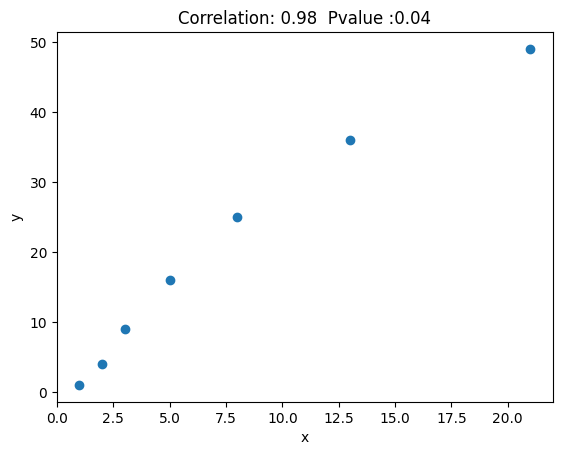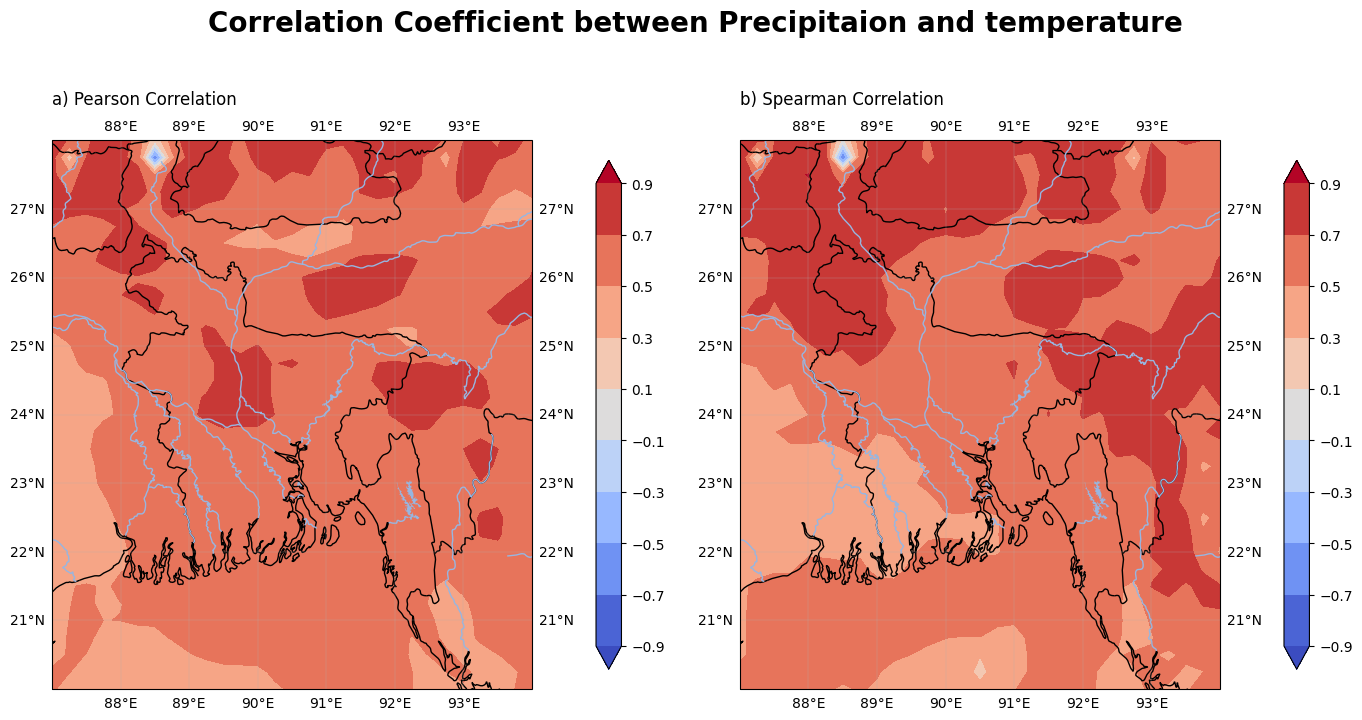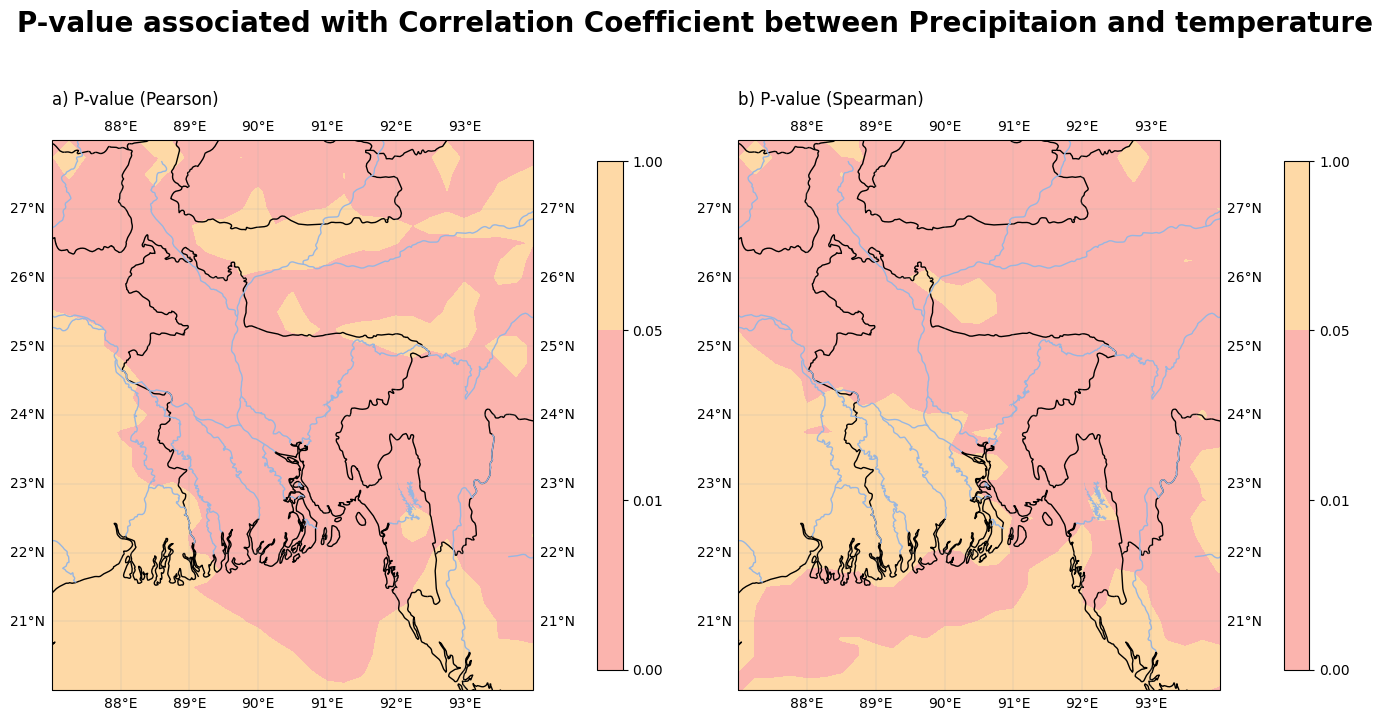# Authors:¶

In :
# from google.colab import drive
# drive.mount('/content/drive')

In [ ]:
!pip uninstall -y shapely
!pip install cartopy==0.21
!pip install xskillscore

In :


In :
import numpy as np
import matplotlib.pyplot as plt
import cartopy.crs as ccrs
import cartopy.feature as cf
import xarray as xr
import xskillscore as xs
import requests


## Correlation¶

Correlation is a statistical measure that indicates the strength and direction of a relationship between two variables. It ranges from -1 to 1, where -1 represents a perfect negative correlation, 0 indicates no correlation, and 1 indicates a perfect positive correlation.

In :
x = [1, 2, 3, 5, 8, 13, 21]
y = [1, 4, 9, 16, 25, 36, 49]

corr = np.corrcoef(x, y)[0, 1]
pvalue = 2 * (1 - np.abs(round(corr, 2))) #ref
print( corr)
print(pvalue)

0.9846915385844243
0.040000000000000036


To find the correlatin coefficient between 'x' and 'y', we use np.corrcoef() function. We will get a matrix or pearson correlation coefficients, as we pass 2 arrays we will get 2x2 matrix in which correlation coefficient between 'x' and 'x' is top left corner or array position [0, 0] and the correlation coefficiant between 'x' and 'y' is top right corner or array position is [0, 1] which is approximately 0.93.

Now, the p-value is the stastical significance of the correlation coefficient. In general, a p-value less than 0.05 is considered significant, which means that there is less than a 5% chance that the correlation is due to chance.

In :
fig, ax = plt.subplots()
ax.scatter(x, y)
ax.set_xlabel('x')
ax.set_ylabel('y')
ax.set_title('Correlation: ' + str(np.around(corr.min(),2))+ '  Pvalue :'+ str(np.around(pvalue,2)))
plt.show()
#draw trendlineWe specify the URL in the file_url variable.

Next, we make a request to the URL using requests.get(). The response object contains the content of the file.

We then open a file in binary write mode ("wb") at the desired location ("/content/data.nc") and write the content of the response into it.

Finally, we use xr.open_dataset() from xarray to open the downloaded file and load it into the data variable. You can replace "/content/data.nc" with your desired file path.

After executing this code, we have the data loaded into the data variable, and proceed with further analysis or operations using xarray.

In :
file_url = "https://drive.google.com/uc?id=10Cf3CbMwX6Z2hnIZg8wWDVajhm6rZ2OU&export=download"
file_path = "/content/data.nc"

response = requests.get(file_url)
with open(file_path, "wb") as f:
f.write(response.content)

data

Out:
<xarray.Dataset>
Dimensions:    (longitude: 29, latitude: 33, time: 8760)
Coordinates:
* longitude  (longitude) float32 87.0 87.25 87.5 87.75 ... 93.5 93.75 94.0
* latitude   (latitude) float32 28.0 27.75 27.5 27.25 ... 20.5 20.25 20.0
* time       (time) datetime64[ns] 2022-01-01 ... 2022-12-31T23:00:00
Data variables:
t2m        (time, latitude, longitude) float32 253.7 253.5 ... 286.0 284.4
tp         (time, latitude, longitude) float32 1.02e-05 2.856e-05 ... 0.0
Attributes:
Conventions:  CF-1.6
history:      2023-07-04 14:04:14 GMT by grib_to_netcdf-2.25.1: /opt/ecmw...
In :
precipitation = data['tp'] * 1000
temperature = data['t2m']

temperature=temperature.resample(time="1M").mean()
precipitation=precipitation.resample(time="1M").mean()
lon = data['longitude']
lat = data['latitude']


The two types of correlation mentioned in the code are:

• Pearson Correlation: It measures the linear relationship between two continuous variables. It is sensitive to outliers and assumes that the variables are normally distributed.

• Spearman Correlation: It assesses the monotonic relationship between two variables, which means it captures both linear and non-linear relationships. It is less sensitive to outliers and does not require the variables to be normally distributed. Instead, it relies on rank ordering the data.

In :
# Calculate Pearson correlation coefficient and p-value for 'precipitation' and 'temperature' along the 'time' dimension
corre1 = xs.pearson_r(precipitation, temperature, dim='time')
p_value1 = xs.pearson_r_p_value(precipitation, temperature, dim='time')

# Calculate Spearman correlation coefficient and p-value for 'precipitation' and 'temperature' along the 'time' dimension
corre2 = xs.spearman_r(precipitation, temperature, dim='time')
p_value2 = xs.spearman_r_p_value(precipitation, temperature, dim='time')

# Store the calculated correlation coefficients in a list
corr = [corre1.data, corre2.data]

# Titles for the correlation coefficients, indicating the corresponding method used ('Pearson' or 'Spearman')
corr_title = ["a) Pearson Correlation", "b) Spearman Correlation"]

# Store the calculated p-values in a list
p_value = [p_value1, p_value2]

# Titles for the p-values, indicating the corresponding method used ('Pearson' or 'Spearman')
p_value_title = ["a) P-value (Pearson)", "b) P-value (Spearman)"]

In :
# Create the subplots
fig, axs = plt.subplots(nrows=1, ncols=2, figsize=(14, 8),
subplot_kw={'projection': ccrs.PlateCarree()})

# Plot Pearson and Spearman Correlation between Precipitation and temperature on the subplots
for i, ax in enumerate(axs.flat):
cs = ax.contourf(lon, lat, corr[i], 30, cmap='coolwarm', extend='both', transform=ccrs.PlateCarree(), levels=np.arange(-.9,0.91,.2))
ax.set_extent([87, 94, 20, 28])
bx = ax.gridlines(draw_labels=True, linewidth=0.2)

# Add vertical colorbar to each subplot with a custom size
cbar = plt.colorbar(cs, ax=ax, orientation='vertical', pad=0.1, shrink=0.7)
cbar.ax.tick_params(labelsize=10)

# Add a title to the figure
fig.suptitle('Correlation Coefficient between Precipitaion and temperature', fontsize=20, fontweight='bold')

# Adjust the spacing between subplots and display the plot
plt.tight_layout()

plt.show()In :
# Create the subplots
fig, axs = plt.subplots(nrows=1, ncols=2, figsize=(14, 8),
subplot_kw={'projection': ccrs.PlateCarree()})

# Plot Pearson and Spearman Correlation P-value on the subplots
for i, ax in enumerate(axs.flat):
cs = ax.contourf(lon, lat, p_value[i], 30,  transform=ccrs.PlateCarree(),levels=np.array([0,.01,0.05,1]),cmap='Pastel1')
ax.set_extent([87, 94, 20, 28])
bx = ax.gridlines(draw_labels=True, linewidth=0.2)

# Add vertical colorbar to each subplot with a custom size
cbar = plt.colorbar(cs, ax=ax, orientation='vertical', pad=0.1, shrink=0.7)
cbar.ax.tick_params(labelsize=10)
# cbar.ax.set_title('mm/day', fontsize=10)

# Add a title to the figure
fig.suptitle('P-value associated with Correlation Coefficient between Precipitaion and temperature', fontsize=20, fontweight='bold')

# Adjust the spacing between subplots and display the plot
plt.tight_layout()

plt.show()In :


In :